# Functors, Applicatives, And Monads In Pictures

updated: May 20, 2013

Here's a simple value: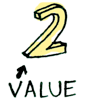And we know how to apply a function to this value:Simple enough. Lets extend this by saying that any value can be in a context. For now you can think of a context as a box that you can put a value in: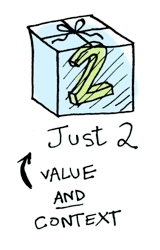Now when you apply a function to this value, you'll get different results depending on the context. This is the idea that Functors, Applicatives, Monads, Arrows etc are all based on. The Maybe data type defines two related contexts:data Maybe a = Nothing | Just a


In a second we'll see how function application is different when something is a Just a versus a Nothing. First let's talk about Functors!

## Functors

When a value is wrapped in a context, you can't apply a normal function to it: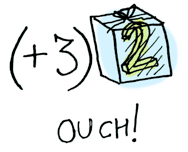This is where fmap comes in. fmap is from the street, fmap is hip to contexts. fmap knows how to apply functions to values that are wrapped in a context. For example, suppose you want to apply (+3) to Just 2. Use fmap:

> fmap (+3) (Just 2)
Just 5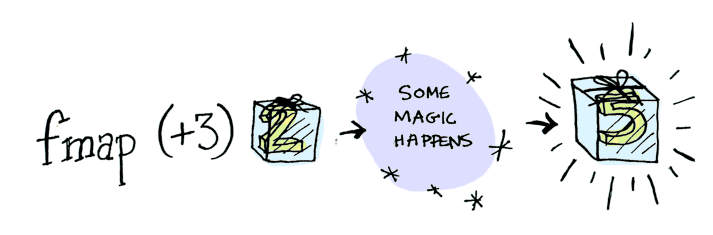Bam! fmap shows us how it's done! But how does fmap know how to apply the function?

## Just what is a Functor, really?

Functor is a typeclass. Here's the definition: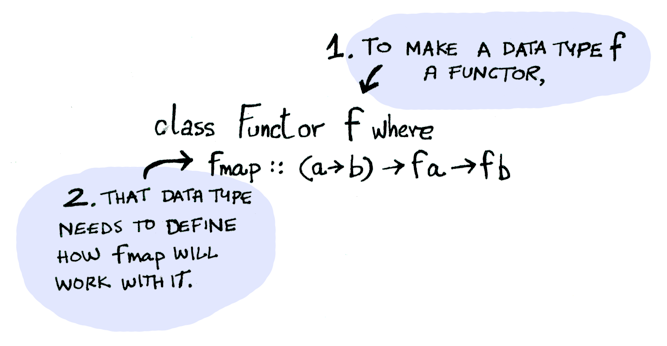A Functor is any data type that defines how fmap applies to it. Here's how fmap works: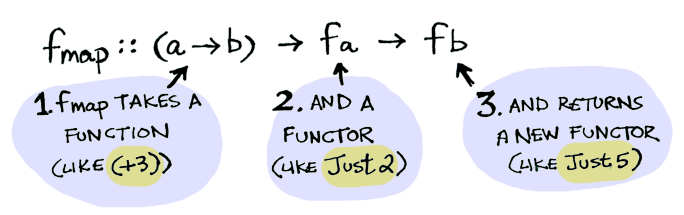So we can do this:

> fmap (+3) (Just 2)
Just 5


And fmap magically applies this function, because Maybe is a Functor. It specifies how fmap applies to Justs and Nothings:

instance Functor Maybe where
fmap func (Just val) = Just (func val)
fmap func Nothing = Nothing


Here's what is happening behind the scenes when we write fmap (+3) (Just 2):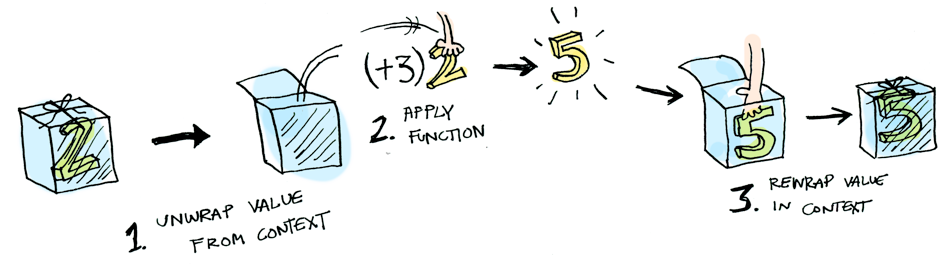So then you're like, alright fmap, please apply (+3) to a Nothing?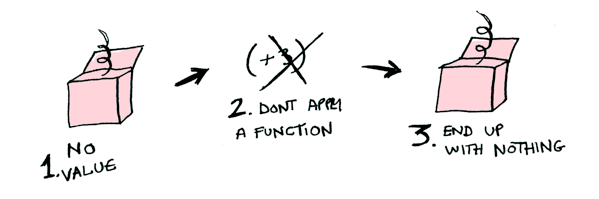> fmap (+3) Nothing
NothingLike Morpheus in the Matrix, fmap knows just what to do; you start with Nothing, and you end up with Nothing! fmap is zen. Now it makes sense why the Maybe data type exists. For example, here's how you work with a database record in a language without Maybe:

post = Post.find_by_id(1)
if post
return post.title
else
return nil
end


But in Haskell:

fmap (getPostTitle) (findPost 1)


If findPost returns a post, we will get the title with getPostTitle. If it returns Nothing, we will return Nothing! Pretty neat, huh? <$> is the infix version of fmap, so you will often see this instead: getPostTitle <$> (findPost 1)


Here's another example: what happens when you apply a function to a list?Lists are functors too! Here's the definition:

instance Functor [] where
fmap = map


Okay, okay, one last example: what happens when you apply a function to another function?

fmap (+3) (+1)


Here's a function: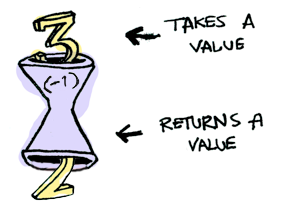Here's a function applied to another function: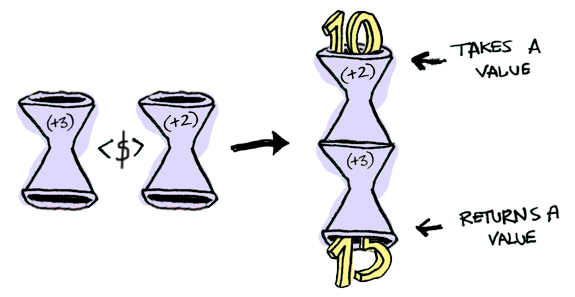The result is just another function!

> import Control.Applicative
> let foo = fmap (+3) (+2)
> foo 10
15


So functions are Functors too!

instance Functor ((->) r) where
fmap f g = f . g


When you use fmap on a function, you're just doing function composition!

## Applicatives

Applicatives take it to the next level. With an applicative, our values are wrapped in a context, just like Functors:But our functions are wrapped in a context too!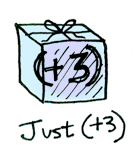Yeah. Let that sink in. Applicatives don't kid around. Control.Applicative defines <*>, which knows how to apply a function wrapped in a context to a value wrapped in a context: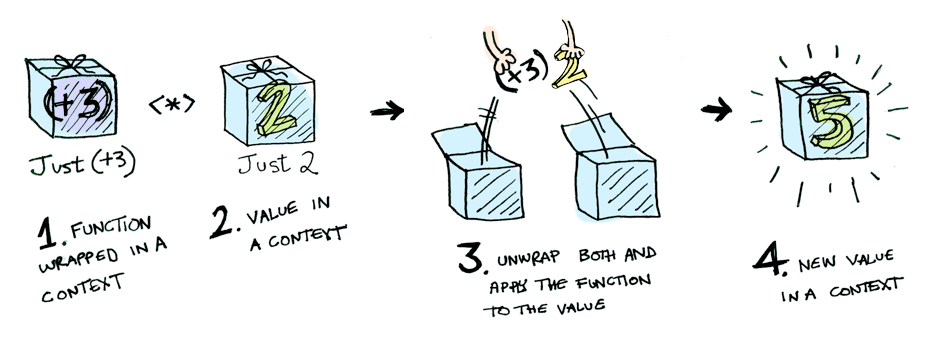i.e:

Just (+3) <*> Just 2 == Just 5


Using <*> can lead to some interesting situations. For example:

> [(*2), (+3)] <*> [1, 2, 3]
[2, 4, 6, 4, 5, 6]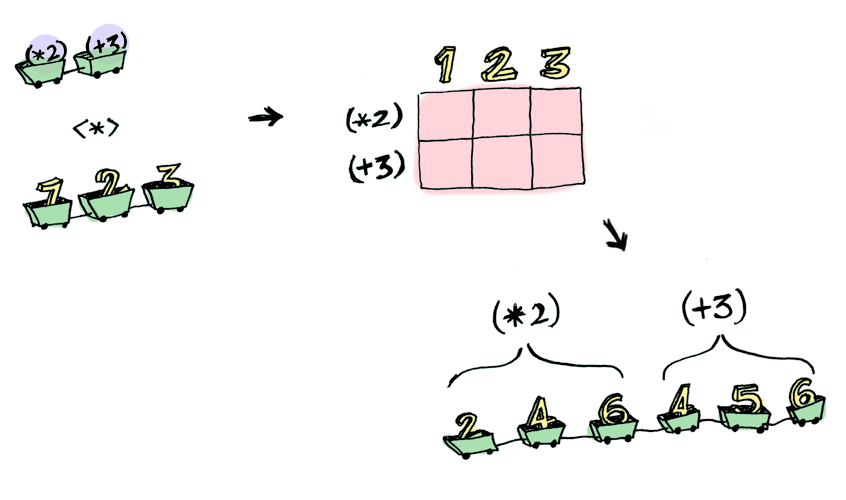Here's something you can do with Applicatives that you can't do with Functors. How do you apply a function that takes two arguments to two wrapped values?

> (+) <$> (Just 5) Just (+5) > Just (+5) <$> (Just 4)
ERROR ??? WHAT DOES THIS EVEN MEAN WHY IS THE FUNCTION WRAPPED IN A JUST


Applicatives:

> (+) <$> (Just 5) Just (+5) > Just (+5) <*> (Just 3) Just 8  Applicative pushes Functor aside. "Big boys can use functions with any number of arguments," it says. "Armed <$> and <*>, I can take any function that expects any number of unwrapped values. Then I pass it all wrapped values, and I get a wrapped value out! AHAHAHAHAH!"

> (*) <$> Just 5 <*> Just 3 Just 15  And hey! There's a function called liftA2 that does the same thing: > liftA2 (*) (Just 5) (Just 3) Just 15  ## Monads How to learn about Monads: 1. Get a PhD in computer science. 2. Throw it away because you don't need it for this section! Monads add a new twist. Functors apply a function to a wrapped value: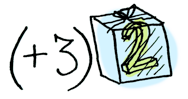Applicatives apply a wrapped function to a wrapped value: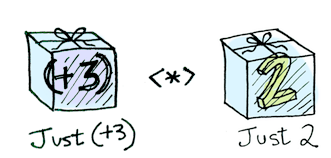Monads apply a function that returns a wrapped value to a wrapped value. Monads have a function >>= (pronounced "bind") to do this. Let's see an example. Good ol' Maybe is a monad:Suppose half is a function that only works on even numbers: half x = if even x then Just (x div 2) else Nothing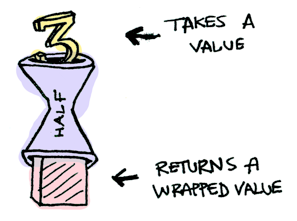What if we feed it a wrapped value?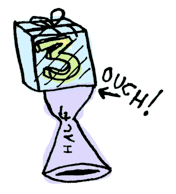We need to use >>= to shove our wrapped value into the function. Here's a photo of >>=:Here's how it works: > Just 3 >>= half Nothing > Just 4 >>= half Just 2 > Nothing >>= half Nothing  What's happening inside? Monad is another typeclass. Here's a partial definition: class Monad m where (>>=) :: m a -> (a -> m b) -> m b  Where >>= is: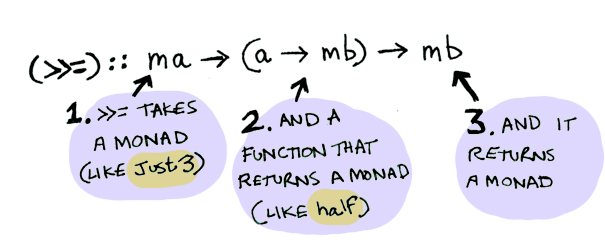So Maybe is a Monad: instance Monad Maybe where Nothing >>= func = Nothing Just val >>= func = func val  Here it is in action with a Just 3!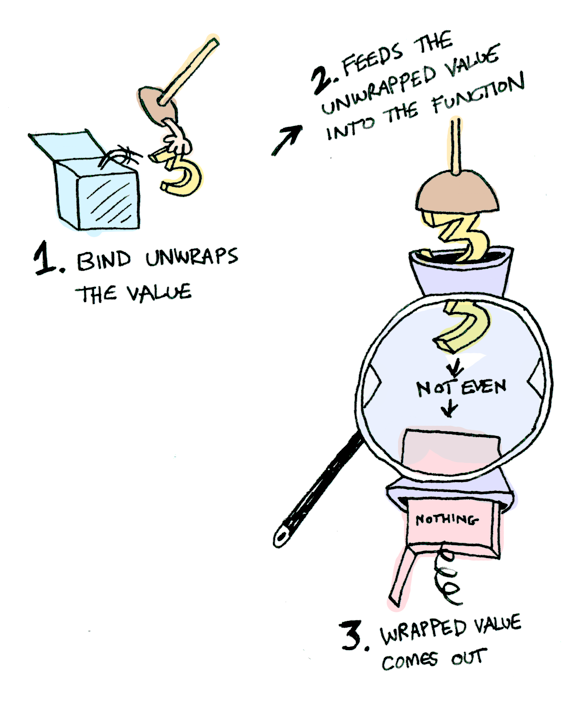And if you pass in a Nothing it's even simpler: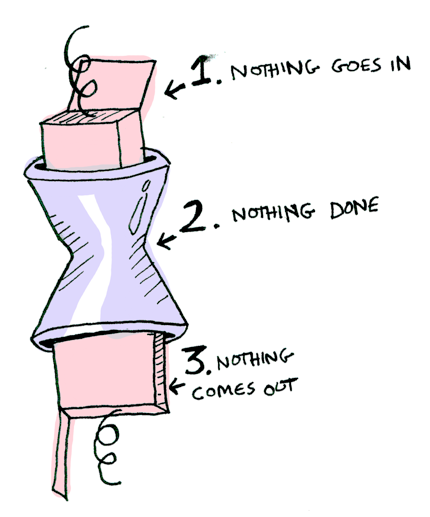You can also chain these calls: > Just 20 >>= half >>= half >>= half Nothing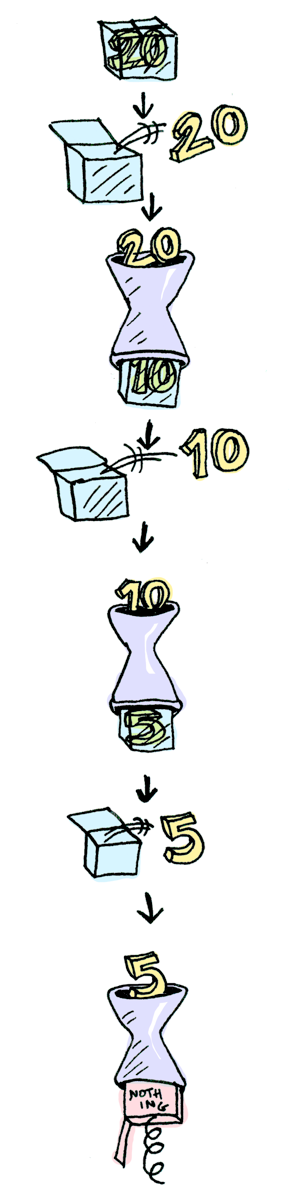Cool stuff! So now we know that Maybe is a Functor, an Applicative, and a Monad. Now let's mosey on over to another example: the IO monad:Specifically three functions. getLine takes no arguments and gets user input:getLine :: IO String  readFile takes a string (a filename) and returns that file's contents: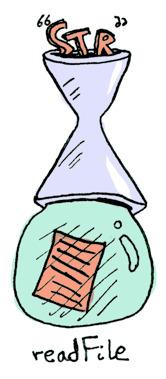readFile :: FilePath -> IO String  putStrLn takes a string and prints it:putStrLn :: String -> IO ()  All three functions take a regular value (or no value) and return a wrapped value. We can chain all of these using >>=!getLine >>= readFile >>= putStrLn  Aw yeah! Front row seats to the monad show! Haskell also provides us with some syntactical sugar for monads, called do notation: foo = do filename <- getLine contents <- readFile filename putStrLn contents  ## Conclusion 1. A functor is a data type that implements the Functor typeclass. 2. An applicative is a data type that implements the Applicative typeclass. 3. A monad is a data type that implements the Monad typeclass. 4. A Maybe implements all three, so it is a functor, an applicative, and a monad. What is the difference between the three?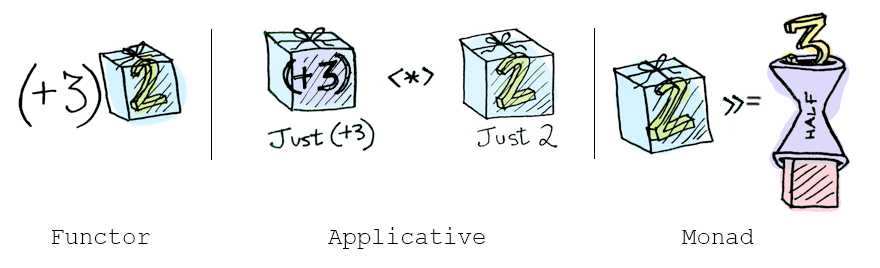• functors: you apply a function to a wrapped value using fmap or <$>
• applicatives: you apply a wrapped function to a wrapped value using <*> or liftA
• monads: you apply a function that returns a wrapped value, to a wrapped value using >>= or liftM

So, dear friend (I think we are friends by this point), I think we both agree that monads are easy and a SMART IDEA(tm). Now that you've wet your whistle on this guide, why not pull a Mel Gibson and grab the whole bottle. Check out LYAH's section on Monads. There's a lot of things I've glossed over because Miran does a great job going in-depth with this stuff.

## Translations

This post has been translated into:

Human languages:

Programming languages:

If you translate this post, send me an email and I'll add it to this list!

For more monads and pictures, check out three useful monads.